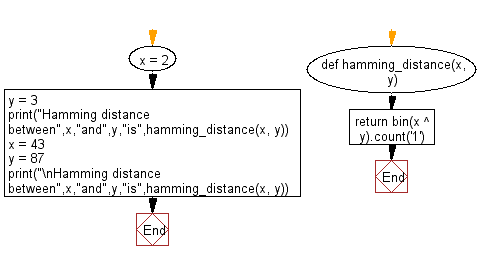﻿ Python Math: Calculate the Hamming distance between two given values - w3resource# Python Math: Calculate the Hamming distance between two given values

## Python Math: Exercise-86 with Solution

In information theory, the Hamming distance between two strings of equal length is the number of positions at which the corresponding symbols are different. In other words, it measures the minimum number of substitutions required to change one string into the other, or the minimum number of errors that could have transformed one string into the other. In a more general context, the Hamming distance is one of several string metrics for measuring the edit distance between two sequences. It is named after the American mathematician Richard Hamming.

Write a Python program to calculate the Hamming distance between two given values.

• Use the XOR operator (^) to find the bit difference between the two numbers.
• Use bin() to convert the result to a binary string.
• Convert the string to a list and use count() of str class to count and return the number of 1s in it.

Sample Solution:

Python Code:

``````def hamming_distance(x, y):
return bin(x ^ y).count('1')
x = 2
y = 3
print("Hamming distance between",x,"and",y,"is",hamming_distance(x, y))
x = 43
y = 87
print("\nHamming distance between",x,"and",y,"is",hamming_distance(x, y))
```
```

Sample Output:

```Hamming distance between 2 and 3 is 1

Hamming distance between 43 and 87 is 5
```

Flowchart:## Visualize Python code execution:

The following tool visualize what the computer is doing step-by-step as it executes the said program:

Python Code Editor:

Have another way to solve this solution? Contribute your code (and comments) through Disqus.

What is the difficulty level of this exercise?

Test your Programming skills with w3resource's quiz.

﻿

```>>> students = [{'name': 'John', 'score': 98}, {'name': 'Mike', 'score': 94}, {'name': 'Jennifer', 'score': 99}]